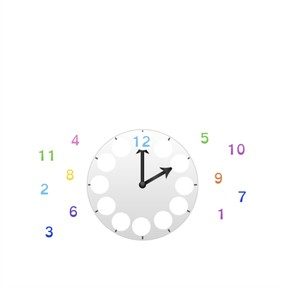Introduction to telling time with analog clocks

# Introduction to telling time with analog clocks

I understand how to tell time on analog clocks.

No account needed.8,000 schools use Gynzy92,000 teachers use Gynzy1,600,000 students use Gynzy

## General

Students are introduced to telling time on the analog clock and work on telling time in whole hours.

## Standards

CCSS.MATH.CONTENT.1.MD.B.3

## Learning objective

Students will be able to tell time on analog clocks.

## Introduction

Discuss a few questions as a class. Where do they see clocks? Which clocks do they have at home? What time do they leave to go to school? What time do they go to bed?

## Instruction

Start by discussing the importance of being able to tell time in whole hours on the analog clock. Explain that the 12 is always at the top of the analog clock. Check that students understand where the numbers belong by having them drag the numbers to where they belong on the clock. Then explain how the hands on the clock work. There are two hands on a clock: the big, or minute hand and the little, or hour hand. The big hand tells us how many minutes have passed and the little hand tells us how many hours. If the big hand is on the 12 it is a whole hour. Show the clock face with the minute hand on 12 and the hour hand on 1. Tell students that it is now one o' clock. Show students the clock with both hands pointing at 12. Show that as the big hand completes a rotation, the small hand moves forward. It is now 1 o'clock. Use the following questions to check that students understand how to tell time. Have them explain how to tell time. Then ask students to drag the clocks into correct order, starting at the given time. For example,: On the left side is the clock that reads 1 o'clock. Then comes the clock that reads 3 o'clock, followed by 4, 6, and 7 o'clock. Students are then given the task to drag the correct clock to the given story.

Check that students are able to tell time on an analog clock by asking the following questions:
- Where is the 12 on a clock?
- Where is the big (minute) hand when it says that it is a whole hour?
- Why is it useful to be able to tell time?

## Quiz

Students are given a set of 10 questions in which they must say what time it is, drag clocks into order, and match clocks with a given story.

## Closing

Discuss the importance of being able to tell time on the analog clock, so they can tell what time it is. To close the lesson, have students tell the time on the given clocks.

## Teaching tips

Students who have difficulty with this learning goal can be supported by making use of an instruction-clock. Re-teach the significance of the big (minute) and little (hour) hands and show how the hour hand moves slowly as the minute hand travels around in a circle. Students can also work in pairs to move the hour hand, creating whole hours on the instruction clock and then have their partner tell them what time it is, what the next hour would be, or what the previous hour was.

## Instruction materials

Instruction clocks

### The online teaching platform for interactive whiteboards and displays in schools

• Save time building lessons

• Manage the classroom more efficiently

• Increase student engagement